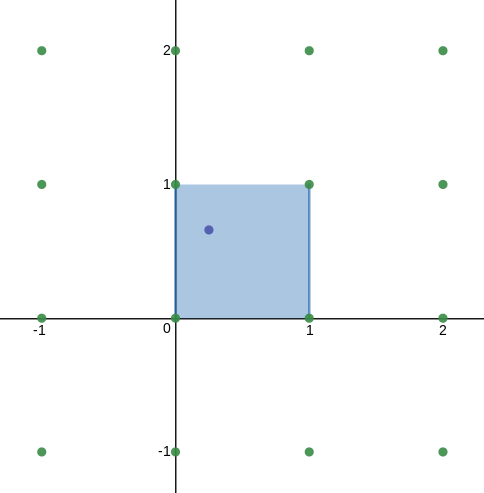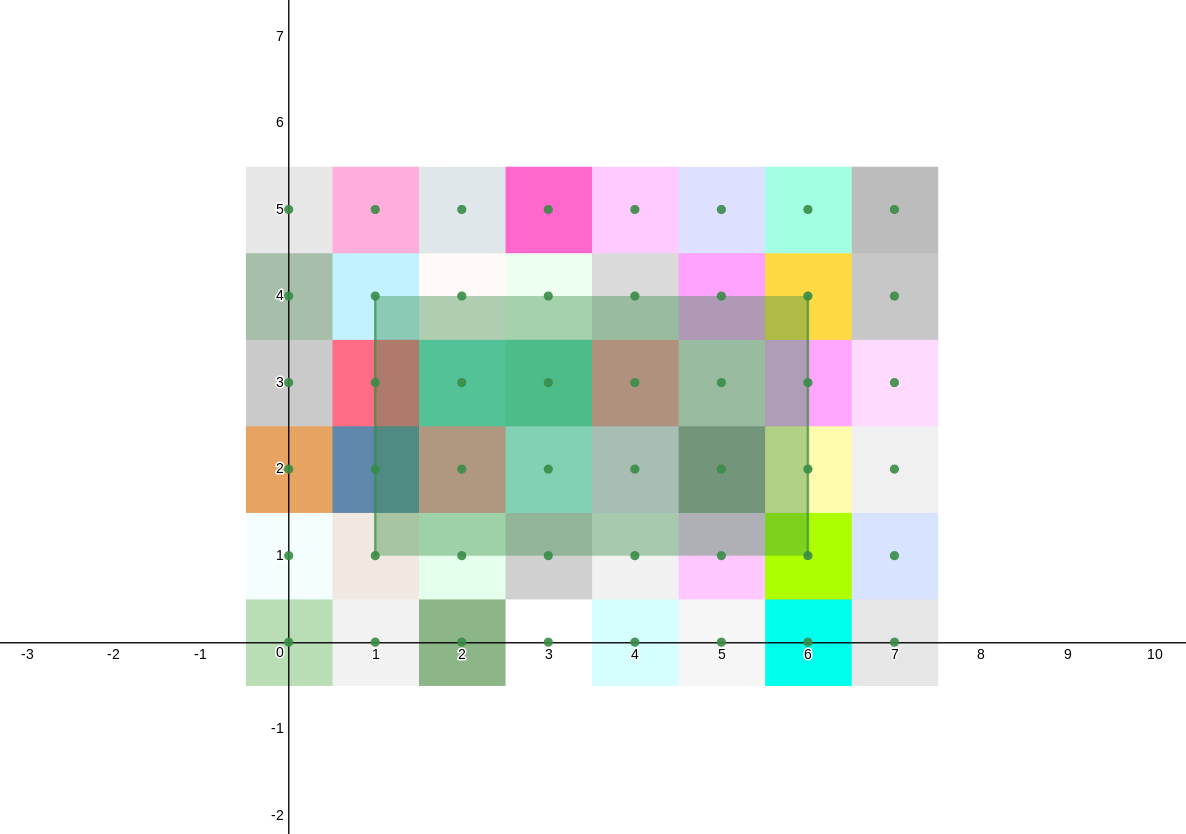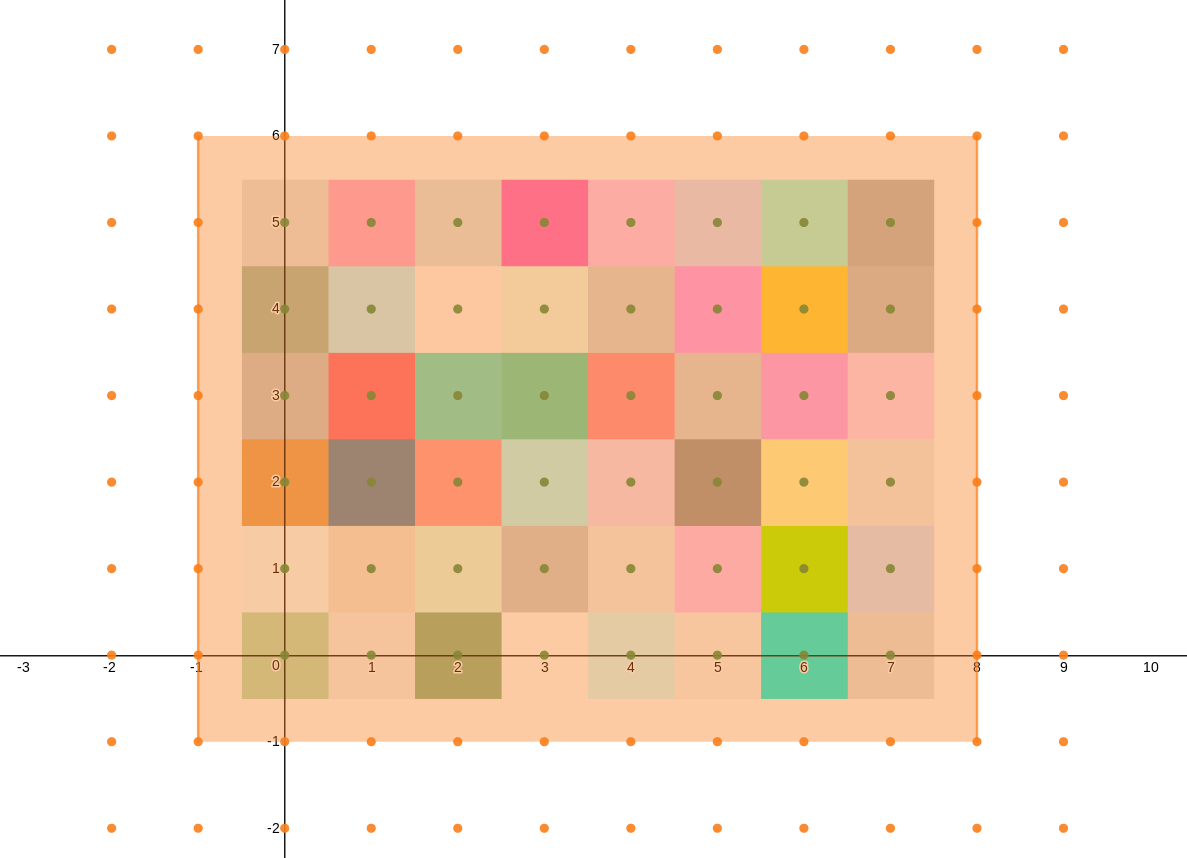# bicubic-interpolate1.0.4 • Public • Published

# bicubic-interpolate

A javascript library for interpolating 2D scalar fields/ 3D surfaces.

• Arbitrary interpolated points - Interpolate whatever point you want, the library doesn't just add points with regular spacings.
• Caching - The coefficients are cached, making significant numbers of calls to the interpolator more efficient.
• Easy tiling/ grid - Automatically creates and caches a grid of individual bicubic interpolators, estimates data points outside the provided region (allowing the interpolation to work to the edge of your data and beyond, if poorly).
• Scaling and Translating - Have the interpolator scale and translate your points so that you don't have to manually convert between your coordinate space and the interpolator's every time.
• Interpolate an arbitrary number of surfaces - Easily interpolate multiple scalar fields in the same region (e.g. RGB components of an image).
• Pre-bundled - Ready to drop in to vanilla javascript or web woker environments.
• Adopter of the Contributor Covenant - This is my first open source project/ library that I'm really intending to be maintained and usable by the community. Though it's a small project, I think the ideas behind the Contributor Covenant are great and the repository will be maintained as described in the Code of Conduct. Please contact me at me@davidbol.es to report any potentially violating behavior or with any questions.
• Released under BSD0, a.k.a the "Free Public License" - Use this code for anything you like and don't worry about having to include it's license. Just don't blame me if anything goes wrong!

# Drawbacks

• Testing - Currently this project includes no automated testing and hasn't even been rigorously tested manually. I'm using it for my own project so major problems should get fixed quickly but subtle issues might be missed. Contributions welcome!
• Performance - This library is generally optimized for my needs (doing lots of interpolation over a large grid) but its caching might actually slow down other applications (e.g. scaling images down).
• Only Bicubic - Only bicubic interpolation (as opposed to ncubic or other interpolation methods) is currently supported. I'd be happy for this project to extend beyond its current scope if someone wants to contribute more algorithms though!

# Docs

## Setup

### Node.js

Install it as normal:

npm i bicubic-interpolate


and then require() it:

const bicubic = require('bicubic-interpolate');


### Web

Include the following (generally in your <head> section):

<script src="https://unpkg.com/bicubic-interpolate@^1.0.0/dist/min.js"></script>


### Web Workers

Simply use importScripts():

importScripts('https://unpkg.com/bicubic-interpolate@^1.0.0/dist/min.js');


## Background

Bicubic interpolation guesses the values for a function of the form f(x, y) = z at a 2D point (purple) that is within a unit square (blue) by considering a 4x4 grid of samples around it (green):You can then make a grid of these interpolators to, for instance, interpolate over an image:To be able to use bicubic interpolation at or past the edges of a grid of samples, additional sample points have to be estimated (this library uses linear extrapolation):## Usage

### createInterpolator(values [, options])

Create a bicubic interpolator function.

Arguments:

• values - A 2D array corresponding to the 16 samples needed to interpolate within the square from (0, 0) to (1, 1). values[i][j] should equal f(i-1, j-1) ( does NOT correspond to (0, 0)!), this is rotated from how they appear if defined in javascript manually.
• options (optional) - An object containing configuration options for the interpolator.
• scaleX and scaleY - Multiply the x/y values input to the interpolator by before interpolating. Default: 1
• translateX and translateY - Add to the x/y values input to the interpolator by before interpolating but after scaling. Default: 0

Returns: A function that takes in x and y values between 0 and 1 as its two arguments and returns an estimated value for z.

Examples:

const interpolator = bicubic.createInterpolator([
[1, 2, 4, 8],//x=-1
[2, 4, 8, 16],//x=0
[3, 6, 12, 24],//x=1
[4, 8, 16, 32]//x=2
]);
console.log('@(0, 0)', interpolator(0, 0));//Notice that this is  in the array, not 
console.log('@(1, 0)', interpolator(1, 0));//Notice that this is the 3rd row, not the 3rd column, although (x, y) corresponds to [x, y]
console.log('@(0, 1)', interpolator(0, 1));//Notice that this is the 3rd column, not the 3rd row
console.log('@(1, 1)', interpolator(1, 1));
console.log('@(0.5, 0.5)', interpolator(0.5, 0.5));//Woo! Interpolated!
console.log('@(pi/4, e/3)', interpolator(Math.PI/4, Math.E/3));//And you can interpolate at any point you want.

//Keep both x and y within the 0-1 range or an error will be thrown.
try {
interpolator(-0.1, 2);//But keep both x and y within the 0-1 range or an error will be thrown.
}catch(e) {
console.warn(e);
}

//You can also do some basic transforms to convert points into 'interpolator space' if you're using a different coordinate system.
const transformingInterpolator = bicubic.createInterpolator([
[1, 2, 4, 8],//x=-1
[2, 4, 8, 16],//x=0
[3, 6, 12, 24],//x=1
[4, 8, 16, 32]//x=2
], {
scaleX: 0.5,
scaleY: 1/3,
translateX: -4,
translateY: 0.2
});
console.log('@(8, 2.4) (transforming)', transformingInterpolator( (1 - (-4))/0.5, (1 - 0.2)/(1/3) ));//The coordinate system is transformed such that (8, 2.4) corresponds to the untransformed (1, 1)


### createGridInterpolator(values [, options])

Create a function that interpolates over multiple unit squares.

Arguments:

• values - A 2D array corresponding to the m x n samples needed to interpolate within the (m-3) x (n-3) rectangle from (1, 1) to (m-2, n-2). values[i][j] should equal f(i, j) ( DOES correspond to (0, 0)!), this is rotated from how they appear if defined in javascript manually.
• options (optional) - An object containing configuration options for the interpolator.
• scaleX and scaleY - Multiply the x/y values input to the interpolator by before interpolating. Default: 1
• translateX and translateY - Add to the x/y values input to the interpolator by before interpolating but after scaling. Default: 0
• extrapolate - Linearly extrapolate a margin of 2 samples around those given (the values array is modified!). Increases possible region for interpolating to the the (m+1) x (n+1) rectangle from (-1, -1) to (m, n). Default: false

Returns: A function that takes in x and y values between 1 and n/m - 2 (or -1 and n/m if options.extrapolate is true) as its two arguments and returns an estimated value for z.

Examples:

const gridInterpolator = bicubic.createGridInterpolator([
[1, 2, 4, 8, 16],//x=0
[2, 4, 8, 16, 32],//x=1
[3, 6, 12, 24, 48],//x=2
[4, 8, 16, 32, 64],//x=3
[5, 10, 20, 40, 80],//x=4
[6, 12, 24, 48, 96],//x=5
]);
console.log('@(1, 1)', gridInterpolator(1, 1));//Notice that this is actually  in the array, unlike for the individual interpolator (where it would be )
console.log('@(1.5, 1.5)', gridInterpolator(1.5, 1.5));//Woo! Interpolated!
console.log('@(2.2, 2.7)', gridInterpolator(4, 2.7));//New individual interpolators are automatically created

//Make sure 1 <= x <= m-2 and 1 <= y <= n-2
try {
gridInterpolator(0.9, 3.1);
}catch(e) {
console.warn(e);
}

//Unless you enable extrapolating, in which case you can go out as far as -1 and m/n
console.log('extrapolated interpolating',
bicubic.createGridInterpolator([
[1, 2, 4, 8, 16],//x=0
[2, 4, 8, 16, 32],//x=1
[3, 6, 12, 24, 48],//x=2
[4, 8, 16, 32, 64],//x=3
[5, 10, 20, 40, 80],//x=4
[6, 12, 24, 48, 96],//x=5
], {extrapolate: true})(6, 5)
);

//Grid interpolators can transform your points before interpolating just like individual interpolators:
const transformingGridInterpolator = bicubic.createGridInterpolator([
[1, 2, 4, 8, 16],//x=0
[2, 4, 8, 16, 32],//x=1
[3, 6, 12, 24, 48],//x=2
[4, 8, 16, 32, 64],//x=3
[5, 10, 20, 40, 80]//x=4
], {
scaleX: 0.5,
scaleY: 1/3,
translateX: -4,
translateY: 0.2
});
console.log('@(8, 2.4) (transforming grid)', transformingGridInterpolator( (1 - (-4))/0.5, (1 - 0.2)/(1/3) ));//The coordinate system is transformed such that (8, 2.4) corresponds to the untransformed (1, 1)

//Grid interpolators cache the individual interpolators they use
var start = new Date();
const newGridInterpolator = bicubic.createGridInterpolator([
[1, 2, 4, 8],//x=0
[2, 4, 8, 16],//x=1
[3, 6, 12, 24],//x=2
[4, 8, 16, 32],//x=3
]);
for(var i = 0; i < 1000000; i++) {
newGridInterpolator(1.5, 1.5);
}
console.log('grid interpolate 1 million times:', (new Date()) - start + 'ms');

start = new Date();
for(var i = 0; i < 1000000; i++) {
const newInterpolator = bicubic.createInterpolator([
[1, 2, 4, 8],//x=-1
[2, 4, 8, 16],//x=0
[3, 6, 12, 24],//x=1
[4, 8, 16, 32]//x=2
]);
newInterpolator(0.5, 0.5);
}
console.log('uncached interpolate 1 million times:', (new Date()) - start + 'ms');


### createMultiInterpolator(values [, options]) and createMultiGridInterpolator(values [, options])

These are helper functions for interpolating multiple surfaces/ scalar fields over the same region (e.g. f(x, y) and g(x, y) or RGB components of an image). They work exactly like their corresponding functions except that values is 3D (e.g. values[x][y][surfaceIndex]) and the interpolator returns an array (e.g. [rInterpolation, gInterpolation, bInterpolation]).

Examples:

//Interpolate multiple surfaces at once
const multiInterpolator = bicubic.createMultiInterpolator([
[[1, 32], [2, 24],  [4, 16],  [8, 8]],//x=-1
[[2, 16], [4, 12],  [8, 8],   [16, 4]],//x=0
[[3, 8],  [6, 6],   [12, 4],  [24, 2]],//x=1
[[4, 4],  [8, 3],   [16, 2],  [32, 1]]//x=2
]);
console.log('@(0, 0)', multiInterpolator(0, 0));
console.log('@(1, 1)', multiInterpolator(1, 1));
console.log('@(pi/4, e/3)', multiInterpolator(Math.PI/4, Math.E/3));


## Running Examples

To see what the examples output, either clone the repository and run node examples.js or just run node node_modules/bicubic-interpolate/examples.js from a project that has bicubic-interpolate installed.

## Package Sidebar

### Install

npm i bicubic-interpolate

### Repository

github.com/david476/bicubic-interpolate

32

1.0.4

0BSD

104 kB

11